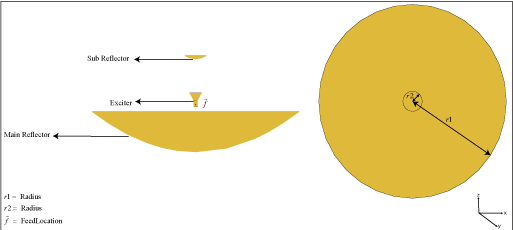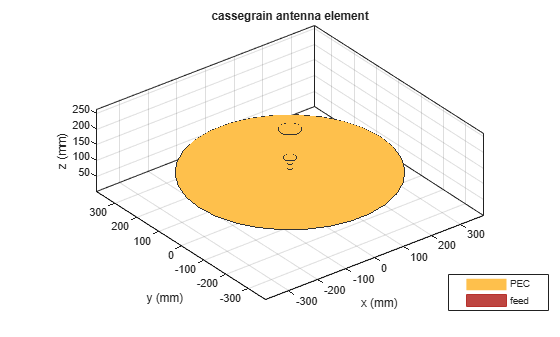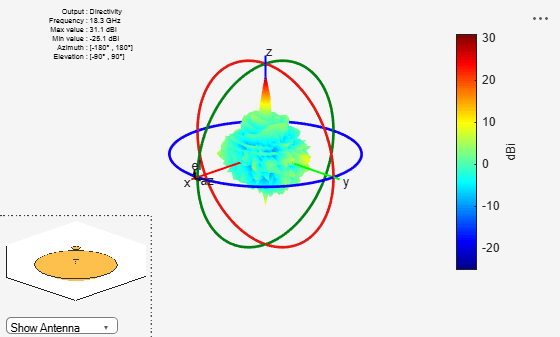# cassegrain

Create Cassegrain antenna

## Description

The `cassegrain` object creates a Cassegrain antenna. A Cassegrain antenna is a parabolic antenna using a dual reflector system. In this antenna, the feed antenna is mounted at or behind the surface of the main parabolic reflector and aimed at the secondary reflector. For more information see, Architecture of Cassegrain Antenna.

Cassegrain antennas are used in applications such as satellite ground-based systems.## Creation

### Syntax

``ant = cassegrain``
``ant = cassegrain(Name,Value)``

### Description

example

````ant = cassegrain` creates a conical horn fed Cassegrain antenna with a resonating frequency of 18.51 GHz. This antenna gives maximum gain when operated at 18 GHz.```
````ant = cassegrain(Name,Value)` sets properties using one or more name-value pairs. For example, `ant = cassegrain('Radius',[0.4 0.22])` creates a Cassegrain antenna with the main reflector with radius 0.4 m and the secondary reflector with radius 0.22 m.```

## Properties

expand all

Antenna type used as exciter, specified as an antenna or an array object.

Example: `'Exciter',dipole`

Example: `ant.Exciter = dipole`

Example: ```ant.Exciter = linearArray('patchMicrostrip')```

Radius of the main and subreflector, specified as a two-element vector with each element unit in meters. The first element specifies the radius of the main reflector, and the second element specifies the radius of the subreflector.

Example: `'Radius',[0.4 0.2]`

Example: `ant.Radius = [0.4 0.2]`

Data Types: `double`

Focal length of the main and subreflector, specified as a two-element vector with each element unit in meters. The first element specifies the focal length of the main reflector and the second element specifies the focal length of the subreflector.

Example: `'FocalLength',[0.35 0.2]`

Example: `ant.FocalLength = [0.35 0.2]`

Data Types: `double`

Lumped elements added to the antenna feed, specified as a lumped element object. You can add a load anywhere on the surface of the antenna. By default, the load is at the feed. For more information, see `lumpedElement`.

Example: `'Load',lumpedelement`. `lumpedelement` is the object for the load created using `lumpedElement`.

Example: ```ant.Load = lumpedElement('Impedance',75)```

Tilt angle of the antenna, specified as a scalar or vector with each element unit in degrees. For more information, see Rotate Antennas and Arrays.

Example: `'Tilt',90`

Example: `ant.Tilt = 90`

Example: `'Tilt',[90 90]`,`'TiltAxis',[0 1 0;0 1 1]` tilts the antenna at 90 degrees about the two axes defined by the vectors.

Note

The `wireStack` antenna object only accepts the dot method to change its properties.

Data Types: `double`

Tilt axis of the antenna, specified as:

• Three-element vector of Cartesian coordinates in meters. In this case, each coordinate in the vector starts at the origin and lies along the specified points on the X-, Y-, and Z-axes.

• Two points in space, each specified as three-element vectors of Cartesian coordinates. In this case, the antenna rotates around the line joining the two points in space.

• A string input describing simple rotations around one of the principal axes, 'X', 'Y', or 'Z'.

Example: `'TiltAxis',[0 1 0]`

Example: `'TiltAxis',[0 0 0;0 1 0]`

Example: `ant.TiltAxis = 'Z'`

Note

The `wireStack` antenna object only accepts the dot method to change its properties.

Data Types: `double`

Solver for antenna analysis, specified as the comma-separated pair consisting of `'SolverType'` and `'MoM-PO'` or `'PO'` (Physical Optics) or `'MoM'` (Method of Moments) or `'FMM'` (Fast Multipole Method).

Example: `'SolverType'`,`'MOM'`

Data Types: `char`

## Object Functions

 `show` Display antenna or array structure; display shape as filled patch `solver` Access FMM solver for electromagnetic analysis `axialRatio` Axial ratio of antenna `beamwidth` Beamwidth of antenna `charge` Charge distribution on metal or dielectric antenna or array surface `current` Current distribution on metal or dielectric antenna or array surface `design` Design prototype antenna or arrays for resonance around specified frequency `EHfields` Electric and magnetic fields of antennas; Embedded electric and magnetic fields of antenna element in arrays `impedance` Input impedance of antenna; scan impedance of array `mesh` Mesh properties of metal or dielectric antenna or array structure `meshconfig` Change mesh mode of antenna structure `optimize` Optimize antenna or array using SADEA optimizer `pattern` Radiation pattern and phase of antenna or array; Embedded pattern of antenna element in array `patternAzimuth` Azimuth pattern of antenna or array `patternElevation` Elevation pattern of antenna or array `rcs` Calculate and plot radar cross section (RCS) of platform, antenna, or array `returnLoss` Return loss of antenna; scan return loss of array `sparameters` Calculate S-parameter for antenna and antenna array objects `vswr` Voltage standing wave ratio of antenna

## Examples

collapse all

Create and view a Cassegrain antenna.

`ant = cassegrain`
```ant = cassegrain with properties: Exciter: [1x1 hornConical] Radius: [0.3175 0.0330] FocalLength: [0.2536 0.1416] Tilt: 0 TiltAxis: [1 0 0] Load: [1x1 lumpedElement] SolverType: 'MoM-PO' ```
`show(ant)`Plot the radiation pattern of the antenna at 18.3 GHz.

`mesh(ant,'maxEdgeLength',14e-3)````figure; pattern(ant,18.3e9)```Create a rectangular array of crossed dipole antennas.

```e = dipoleCrossed('Tilt',90,'TiltAxis',[0 1 0]); arr = rectangularArray('Element',e,'Rowspacing',0.03,'ColumnSpacing',0.03);```

Use the rectangular array `arr` to excite a Cassegrain antenna.

`ant = cassegrain('Exciter',arr)`
```ant = cassegrain with properties: Exciter: [1x1 rectangularArray] Radius: [0.3175 0.0330] FocalLength: [0.2536 0.1416] Tilt: 0 TiltAxis: [1 0 0] Load: [1x1 lumpedElement] SolverType: 'MoM-PO' ```
`show(ant)`expand all

 Dandu, Obulesu. "Optimized Design of Axillary Symmetric Cassegrain Reflector Antenna Using Iterative Local Search Algorithm"

 Balanis, C.A. Antenna Theory: Analysis and Design. 3rd Ed. New York: Wiley, 2005.